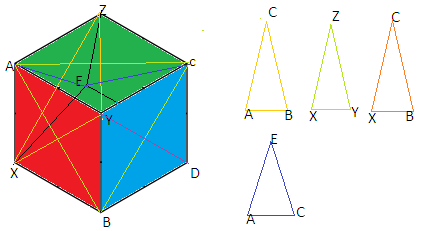QuestionAnswers

# How many triangles whose vertices are chosen from the vertices of a cube are equilateral?A.3 B.2C.4D.8

Hint: A cube has four lateral surfaces, one top surface and one bottom surface. We can get an equilateral triangle by joining the opposite ends of vertices that have a common point which is the point where the cube's angle is formed.Here there are a total four pairs of adjacent lateral surfaces and each pair makes $2$ triangles with bottom and top surfaces.
So Total triangles =$4 \times 2$ =$8$
Now, if we flip or change the bottom and top surfaces then again using the same process we obtain $8$ equilateral triangles. But these triangles will be the same as the first $8$ triangles.
So, total $8$ triangles can be formed when vertices of triangles are chosen from the vertices of a cube.
Note: We can also solve this question by marking the top vertices as A, B, C, D and bottom vertices as W, X, Y, Z. Join diagonal AC then ACX and ACZ are two equilateral triangles. Similarly we can obtain two Triangles by joining each diagonal WY, BZ and XZ. So $2 \times 4 = 8$